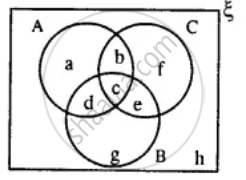# From the Given Diagram, Find : (A ∪ B) - C Verify : a - (B ∩ C) = (A - B) ∪ (A - C) - Mathematics

Diagram

From the given diagram, find :
(i) (A ∪ B) - C

(ii) B - (A ∩ C)

(iii) (B ∩ C) ∪ A

Verify :
A - (B ∩ C) = (A - B) ∪ (A - C)#### Solution

(i) A ∪ B = {a, b, c, d} ∪ {c, d, e, g}

= {a, b, c, d, e, g}

∴ (A ∪ B) - C = {a, b, c, d, e, g} - {b, c, e, f}

= {a, d, g}

(ii)  (A ∩ C) = {a, b, c, d} ∩ {b, c, e, f}

= {b, c}

∴ B - (A ∩ C) = {c, d, e, g} - {b, c}

= {d, e, g}

(iii)  B ∩ C = {c, e, d, g} ∩ {b, c, e, f}

= {c, e}

∴ A - (B ∩ C) = (A - B) ∪ (A - C)

⇒ (B ∩ C) = {c, e}

So, A − (B ∩ C) = {a, b, d}     .....(1)

Now, A − B = {a, b}

And A − C = {a, d}

The union of two sets has the elements of both sets.

So, (A − B) ∪ (A − C) = {a, b, d} .....(2)

From (1) and (2), we have

A − (B ∩ C) = (A − B) ∪ (A − C)

The relation holds True.

Concept: Venn Diagram
Is there an error in this question or solution?

#### APPEARS IN

Selina Concise Mathematics Class 8 ICSE
Chapter 6 Sets
Exercise 6 (E) | Q 10 | Page 76